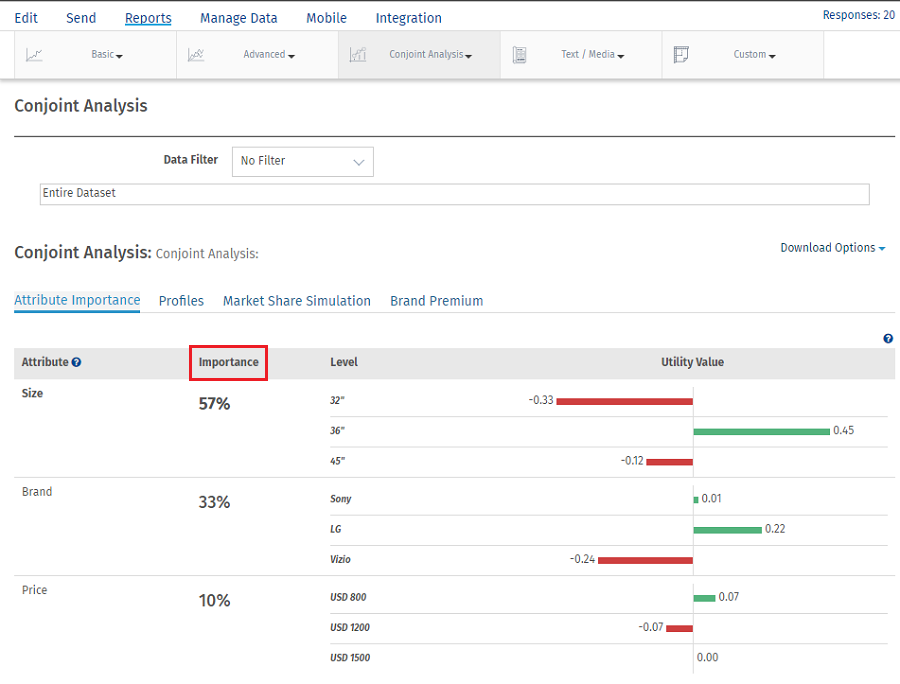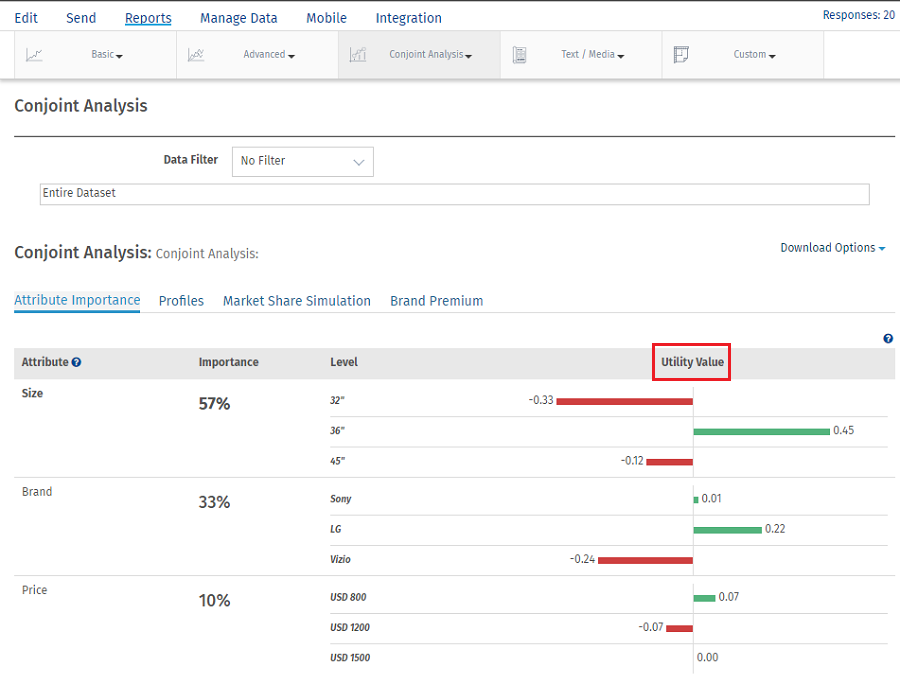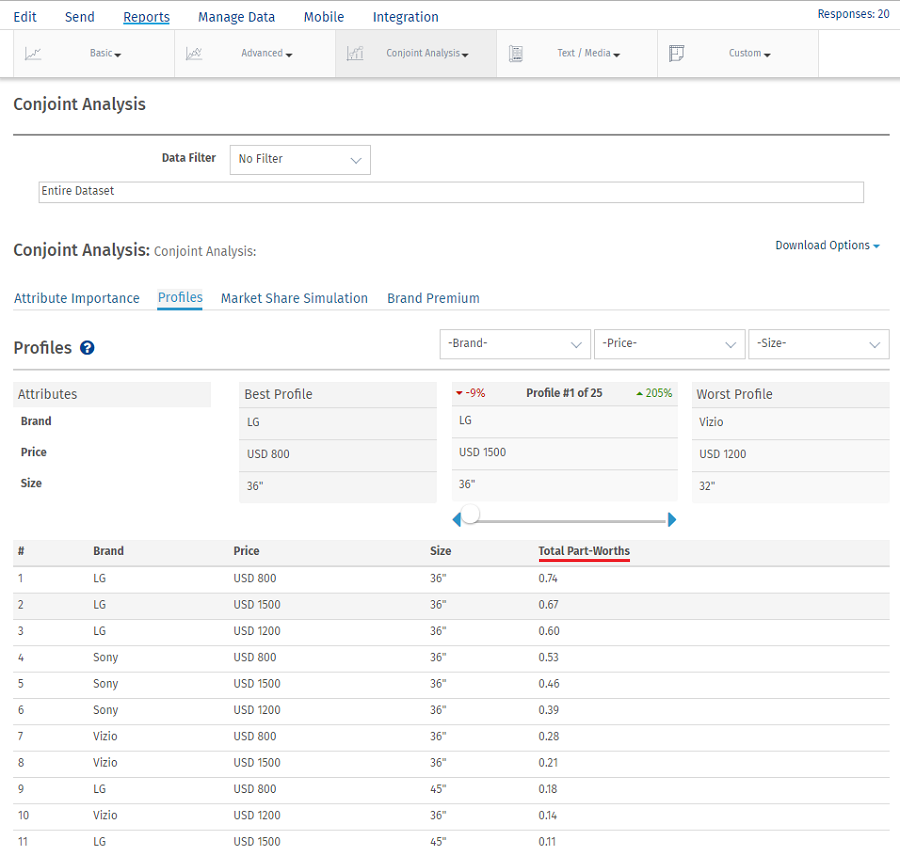# Conjoint Analysis – Attribute Importance

Attribute Importance is also known as Relative Importance, this shows which attributes of a product or service are more or less important when making a purchasing decision.

To view detailed Attribute Importance:

Go to: Login » Surveys » Reports » Choice Modelling » Conjoint Analysis » Attribute ImportanceCalculating Attribute Importance

Sometimes we want to characterize the relative importance of each attribute. We can do this by considering how much difference each attribute could make in the total utility of a product. That difference is the range in the attribute’s utility values.

Importance measures are ratio-scaled,but they are also relative,study-speciﬁc measures. An attribute with an importance of twenty percent is twice as important as an attribute with an importance of ten, given the set of attributes and levels used in the study. That is to say, importance has a meaningful zero point,as do all percentages. But when we compute an attribute’s importance,it is always relative to the other attributes being used in the study. And we can compare one attribute to another in terms of importance within a conjoint study but not across studies featuring different attribute lists.

Example:

Step 1: Calculate Attribute Utility Range

Utility Range = Highest Utility Value of an attribute - Lowest Utility Value of an attribute

For Price Range is calculated as 0.45-(-0.33) = 0.78
For Size Range is calculated as 0.22-(-0.24) = 0.46
For Brand Rage is calculated as 0.07-(-0.07) = 0.14

Step 2 : Calculate Total Attribute Utility Range

Total Utility Range = ∑ Utility Range

Total Attribute Utility Range = 0.78+0.46+0.14 = 1.38

Step 3: Calculating Relative Importance of attributes

Relative Importance of attribute = (Attribute Utility Range/Total Attribute Utility Range)*100

Importance of Price = (0.78/1.38)*100 = 56.52% Rounded off to 57%
Importance of Size = (0.46/1.38)*100 = 33.33% Rounded off to 33%
Importance of Brand = (0.14/1.38)*100 =10.14% Rounded off to 10%

Calculating Utility Value

Conjoint utilities or part-worths are scaled to an arbitrary additive constant within each attribute and are interval data. The arbitrary origin of the scaling within each attribute results from dummy coding in the design matrix. We could add a constant to the part-worths for all levels of an attribute or to all attribute levels in the study, and it would not change our interpretation of the ﬁndings. When using a speciﬁc kind of dummy coding called effects coding, utilities are scaled to sum to zero within each attribute.Utility Value is calculated using part worth value. For every level of attribute summation of part worth is calculated. Then the average of the summation is taken to get the utility value of a particular level.Example: Utility value for size 36” is calculated by taking summation of Total part worths for 36” average of which will give utility value.

Summation of total part worths of 36” = 0.74+0.67+0.60+0.53+0.46+0.39+0.28+0.21+0.14 = 4.02

Average = 4.02/9 which gives utility value of 0.45 for attribute size with level 36’’

This feature/tools described here are available with the following license(s) :

Enterprise Edition

Unlimited Surveys, Questions